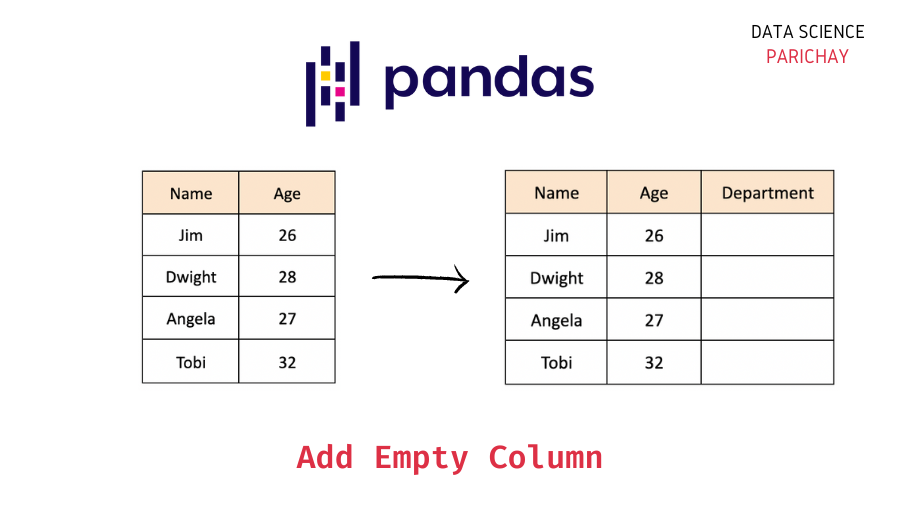# Pandas – Add an Empty Column to a DataFrame

In this tutorial, we will look at how to add an empty column to a pandas dataframe with the help of some examples.

## What is an empty column in a Pandas dataframe?

Depending upon your use-case, you can call a column in a dataframe empty based on a variety of factors. Some of the commonly used criteria for defining an empty column in Pandas are –

• All the values in the column are an empty string, `''`.
• All the values in the column are `NaN`.
• All the values in the column are `None`.

Note that the above list is not exhaustive and it depends on how a particular column gets used. For example, a column that is expected to store string values has all its values as empty strings can be considered an empty column or a numeric column having all values `NaN` maybe considered an empty column.

Thus, it is important to follow the convention (or the guidelines) on empty columns as per your team’s (or project’s) requirements.

## How to add an empty column to a Pandas Dataframe?

In general, there are multiple ways to add a new column to an existing Pandas dataframe. You can use these methods for a special case – adding an empty column to the dataframe.

First, let’s create a sample dataframe that we will be using throughout this tutorial.

```import numpy as np
import pandas as pd

# employee data
data = {
"Name": ["Jim", "Dwight", "Angela", "Tobi"],
"Age": [26, 28, 27, 32]
}

# create pandas dataframe
df = pd.DataFrame(data)

# display the dataframe
df```

Output:

We now have a dataframe containing the name and age information of some employees in an office.

📚 Data Science Programs By Skill Level

Introductory

Intermediate ⭐⭐⭐

🔎 Find Data Science Programs 👨‍💻 111,889 already enrolled

Disclaimer: Data Science Parichay is reader supported. When you purchase a course through a link on this site, we may earn a small commission at no additional cost to you. Earned commissions help support this website and its team of writers.

### Add the empty column at the end of the dataframe

You can initialize the new column and set it to an empty string (or `NaN`, or `None`, depending on the use-case) to add an empty column to a pandas dataframe.

The following is the syntax –

```# add empty column (with empty strings)
df[new_column_name] = ''
# add empty column (with NaNs)
df[new_column_name] = np.nan
# add empty column (with None)
df[new_column_name] = None```

Let’s now add an empty column for the “Department” of these employees. Since we don’t yet have the department information, we’ll set all the values to an empty string. Thus, creating an empty “Department” column in the dataframe.

```# add empty column (with emtpy string as values)
df['Department'] = ''
# display the dataframe
df```

Output:

You can see that a “Deparment” column with empty string values is added at the end of the dataframe.

You can similarly add an empty column with `NaN` or `None` values depending on your use-case.

### Add the empty column at a specific index in the dataframe

To add an empty column at a specific position (column index) in the dataframe, use the pandas dataframe `insert()` function. This function is used to insert a column at a specific index in the dataframe.

We specify, the position to add the new column, the column name and the column values as arguments to the `insert()` function.

Let’s add the empty “Department” column in the original dataframe at the index 1 (as the second column) in the dataframe.

```## create the original dataframe
# employee data
data = {
"Name": ["Jim", "Dwight", "Angela", "Tobi"],
"Age": [26, 28, 27, 32]
}

# create pandas dataframe
df = pd.DataFrame(data)

# insert new empty column (with empty strings as values) at column index 1
df.insert(1, "Department", "")
# display the dataframe
df```

Output:

You can see that the empty “Department” column is added to the dataframe.

Again, you can similarly add columns with `NaN` or `None` values.

Note that the pandas dataframe `insert()` function, modifies the dataframe in place.

## Summary

In this tutorial, we looked at how to add an empty column to a Pandas dataframe. The following are the key takeaways from this tutorial –

• Definition of an empty column (whether it’s a column of empty strings, `NaN`, or `None` values) will depend on your specific use case.
• Number of ways to add an empty column.
• You can initialize a new column and set it to an empty string (or `NaN` or `None`). This will add the empty column to the end of the dataframe.
• Use the pandas dataframe `insert()` function to add an empty column at a specific position (column index) in the dataframe.

You might also be interested in –

•